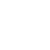Classes (extension) |

# LTI : UGen : AbstractFunction : ObjectExtension

Linear Time Invariant General Filter Equation
Source: SLUGens.sc

SLUGens released under the GNU GPL as extensions for SuperCollider 3, by Nick Collins http://composerprogrammer.com/index.html

## Description

General Linear Time-Invariant (LTI) filter UGen where you specify any coefficient set via a Buffer Represents the general LTI filter difference equation in the time domain:

y(n) = b0x(n) + b1x(n-1) + ... + b(Nb)x(n-Nb) + a1y(n-1) + ... + a(Na)y(n-Na)

This is not a pole/zero view, so you'd need to calculate time domain coefficients yourself if you want to work from z-plane backwards. A corollary is, stability is not guaranteed. This is part of the fun?

You need to pass in the coefficients via two buffers, of arbitrary size.

## Class Methods

### LTI.ar(input, bufnuma: 0, bufnumb: 1, mul: 1.0, add: 0.0)

#### Arguments:

 input What do you want to filter? bufnuma Feedback filter coefficients, from previous outputs bufnumb Feedforward filter coefficients, from previous inputs